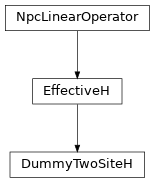# DummyTwoSiteH¶

Inheritance DiagramMethods

 `DummyTwoSiteH.__init__`(*args, **kwargs) Return the hermitian conjugate of self Combine the legs of theta, such that it fits to how we combined the legs of self. Calculate the action of the operator on a vector vec. Contract self to a matrix. `DummyTwoSiteH.update_LP`(env, i[, U]) Equivalent to `env.get_LP(i, store=True)`; optimized for combine. `DummyTwoSiteH.update_RP`(env, i[, VH]) Equivalent to `env.get_RP(i, store=True)`; optimized for combine.

Class Attributes and Properties

 `DummyTwoSiteH.acts_on` `DummyTwoSiteH.length`
class tenpy.algorithms.mps_common.DummyTwoSiteH(*args, **kwargs)[source]

Bases: `EffectiveH`

A dummy replacement for `TwoSiteH()` with similar methods but no actual MPO.

This allows to base the `VariationalCompression` on the `Sweep` class.

combine_theta(theta)[source]

Combine the legs of theta, such that it fits to how we combined the legs of self.

Parameters

theta (`Array`) – Wave function to apply the effective Hamiltonian to, with uncombined legs.

Returns

theta – Wave function with labels as given by self.acts_on.

Return type

`Array`

Return the hermitian conjugate of self

If self is hermitian, subclasses can choose to implement this to define the adjoint operator of self.

matvec(vec)[source]

Calculate the action of the operator on a vector vec.

Note that we don’t require vec to be one-dimensional. However, for square operators we require that the result of matvec has the same legs (in the same order) as vec such that they can be added. Note that this excludes a non-trivial qtotal for square operators.

to_matrix()[source]

Contract self to a matrix.

If self represents an operator with very small shape, e.g. because the MPS bond dimension is very small, an algorithm might choose to contract self to a single tensor.

Returns

matrix – Contraction of the represented operator.

Return type

`Array`

update_LP(env, i, U=None)[source]

Equivalent to `env.get_LP(i, store=True)`; optimized for combine.

Parameters
• env (`MPOEnvironment`) – The same environment as given during class initialization.

• i (int) – We update the part left of site i. Can optimize if i == `i0` and `combine` is True.

• U (None | `Array`) – The tensor on the left-most site self acts on, with combined legs after SVD. Only used if trying to optimize.

update_RP(env, i, VH=None)[source]

Equivalent to `env.get_RP(i, store=True)`; optimized for combine.

Parameters
• env (`MPOEnvironment`) – The same environment as given during class initialization.

• i (int) – We update the part right of site i. Can optimize if i == `i0` + 2 - `length` and `combine` is True.

• U (None | `Array`) – The tensor on the right-most site self acts on, with combined legs after SVD. Only used if trying to optimize.